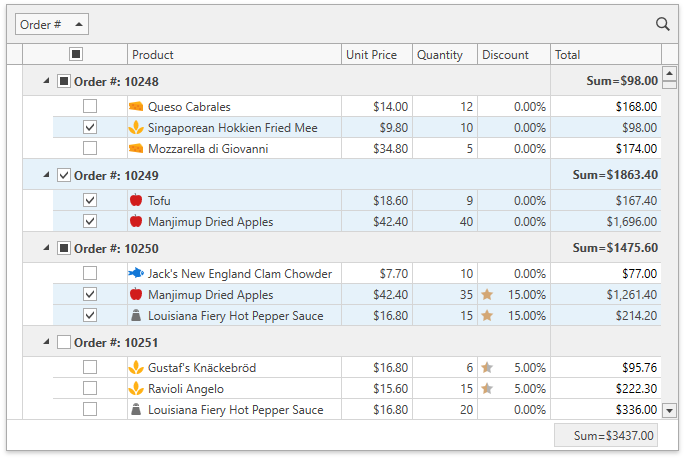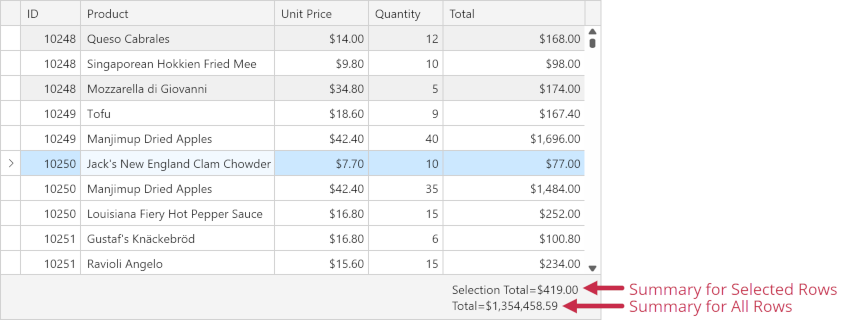20.1
19.2
19.1
18.2
18.1
17.2

# Data Summaries

The GridControl allows you to display summary information about groups of rows or individual data columns. For example, you can display the number of records, the minimum or maximum value, etc. This summary information is called data summary.

## Create a Summary

The GridControl supports Total and Group summaries:

##### TIP

Demos:

The GridSummaryItem objects represent data summaries. You can specify the field against whose values the summary is calculated, the aggregate function, and the summary value format. The GridControl.TotalSummary collection stores Total summaries, the GridControl.GroupSummary collection stores Group summaries.

## Recalculate Summary on Data Updates

The GridControl updates its summary values after you post an edited row's changes to a data source. Call the DataViewBase.CommitEditing method in the GridViewBase.CellValueChanged event handler to update summary values each time a cell value is edited:

``````private void view_CellValueChanged(object sender, DevExpress.Xpf.Grid.CellValueChangedEventArgs e) {
view.CommitEditing();
}
``````

The GridControl does not update its summaries if you modify the control's data source directly (without using the control's UI). Set the DataControlBase.AllowLiveDataShaping property to true to make the control recalculate summaries when the data source changes.

### Optimized Summary Recalculation

The GridControl can use an optimized summary recalculation mechanism, which processes only changed data records if an update is needed. As a result, the time required to update summary values does not depend on the number of records.

Set the GridControl.OptimizeSummaryCalculation property to true to enable the optimized summary recalculation. The GridControl should be bound to an ObservableCollection or ChunkList<T> whose items implement the INotifyPropertyChanged and INotifyPropertyChanging interfaces. The GridControl cannot optimize the recalculation of Custom Summaries and summaries for Unbound Columns.

## Summary for Selection

The GridControl can calculate summaries against selected rows and cells:Set the DataViewBase.SummaryCalculationMode property to SelectedRows / Mixed to make the GridControl calculate all summaries within its view against selected rows.

##### NOTE
• SelectedRows - The summary value is calculated against selected rows.
• AllRows - The summary value is calculated against all rows.
• Mixed - The summary value is calculated against selected rows if their number is more than one; otherwise, against all rows.
``````<dxg:GridControl x:Name="grid" SelectionMode="Row">
<dxg:GridControl.View>
<dxg:TableView x:Name="view"
SummaryCalculationMode="SelectedRows"
ShowTotalSummary="True" />
</dxg:GridControl.View>
<dxg:GridControl.TotalSummary>
<dxg:GridSummaryItem FieldName="Total" SummaryType="Sum" DisplayFormat="Sum={0:\$0.00}" />
</dxg:GridControl.TotalSummary>
<dxg:GridControl.GroupSummary>
<dxg:GridSummaryItem FieldName="Total" SummaryType="Sum" DisplayFormat="Sum={0:\$0.00}" />
</dxg:GridControl.GroupSummary>
</dxg:GridControl>
``````
##### TIP

You can create an individual summary for selected rows/cells and set its SummaryItemBase.CalculationMode property to SelectedRows / Mixed:``````<dxg:GridControl x:Name="grid" SelectionMode="Row">
<!-- -->
<dxg:GridControl.View>
<dxg:TableView x:Name="view" ShowTotalSummary="True" ShowGroupedColumns="True" />
</dxg:GridControl.View>
<dxg:GridControl.TotalSummary>
<!-- The first summary against selected rows -->
<dxg:GridSummaryItem FieldName="Total" SummaryType="Sum" ShowInColumn="Total"
DisplayFormat="Selection Total=\${0:N}" CalculationMode="SelectedRows" />
<!-- The second summary against all rows -->
<dxg:GridSummaryItem FieldName="Total" SummaryType="Sum" ShowInColumn="Total"
DisplayFormat="Total=\${0:N}" />
</dxg:GridControl.TotalSummary>
<dxg:GridControl.GroupSummary>
<dxg:GridSummaryItem FieldName="Total" SummaryType="Sum"
DisplayFormat="Grand Total=\${0:N}" CalculationMode="SelectedRows" />
</dxg:GridControl.GroupSummary>
</dxg:GridControl>
``````